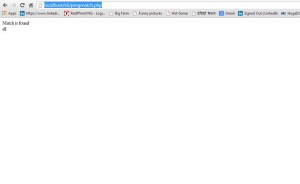Web Programming TutorialsImplementing Conditional and Regular expressions in php

# Implementing Conditional and Regular expressions in php

In this tutorial we will learn to implement the conditional as well as regular expressions in php.

• To study the implementation of conditional and regular expression in php follow the steps given below :

1. CONDITIONAL EXPRESSION
1. Install Xampp server in your machine and if it is already installed then go to Xampp-> htdocs folder .
2. Create a new folder say s6 in this htdocs folder.
3. In this s6 folder create a new file say index.php and open it with notepad++.
4. Now write the given code in index.php file :
5. ```<html>

<body>
<h1>This is the if statement</h1>
<?php

\$x=5;
if(\$x>2)
{
echo  \$x.' is greater than 2';

}

?>
<br />
<h1>This is the if else statement</h1>
<?php

\$x=1;

if(\$x>2)
{
echo  \$x.' is greater than 2';

}
else{
echo  \$x.' is less than 2';

}

?>
<br />
<h1>This is the if elseif statement</h1>
<?php
\$x=5;
if(\$x>2 && \$x<10)
{
echo \$x.' is greater than 2 and less than 10';

}

elseif(\$x==10){
echo \$x.' is equal to 10';
}
else{

echo ' Number is out of bound';
}

?>
<br />
<h1>This is the switch statement</h1>
<?php

\$x=5;
switch(\$x){

case 2:
echo  'The number is 2 ';
break;

case 3:
echo  'The number is 3 ';
break;
default:
echo 'Number is not valid';
}

?>
</body>
</html>
```

6. So you will have the following look out of your notepad++ window :
7. Now start the required modules i.e the Apache and MySql module from the Xampp control pannel .
8. Now open up the browser and enter the url as http://localhost/s6/index.php
9. Hence you will have the following output .

2. REGULAR EXPRESSION
1. Now in the same s6 folder create a new file say pregmatch.php and open it with notepad++ .
2. Now write the following code in it which deals with matching a string :
3. ```<?php
if(preg_match("/ell/","Hellow World",\$matches))
{
echo 'Match is found<br />';
echo \$matches;
}

?>
```

4. So you will have the following view of your notepad++ window :
5. Now open up the browser and enter the given url to run your program http://localhost/s6/pregmatch.php
6. You will have the following output :
7.Note :- if(preg_match(“/ell/”,”Hellow World”,\$matches))
in this condition “/ell/” is used as the find means that is to be found in “Hellow World ” .

Note:- In this condition
if(preg_match(“/^ell/”,”ellow World”,\$matches))
^(caret) symbol represents the beginning string that is starting from “ell” word .If this condition is satisfied then it will display the message Match is found, otherwise it does not display anything in the browser window.

3. Thus we had a brief study about the conditional and regular expressions in php .

### How SEO Impacts Your Sales

21,501Fans
4,210Followers
104,000Subscribers

### How SEO Impacts Your Sales

- Advertisement -